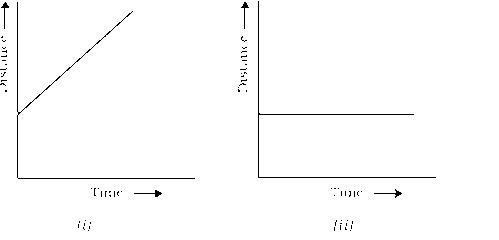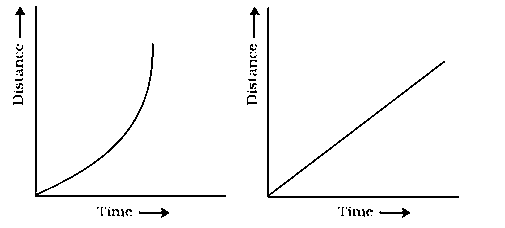### Motion and Time - Solutions

CBSE Class–VII Science

NCERT Solutions
CHAPTER-13
MOTION AND TIME

Question 1. Classify the following as motion along a straight line, circular or oscillatory motion.
(i) Motion of your hands while running.
(ii) Motion of a horse pulling a cart on a straight road.
(iii) Motion of a child in merry-go –round.
(iv) Motion of a child on a see-saw.
(v) Motion of the hammer of an electric bell.
(vi) Motion of a train on a straight bridge.

(i) Oscillatory motion
(ii) Straight line
(iii) Circular motion
(iv) Oscillatory motion
(v) Oscillatory motion
(vi) Straight line.

Question 2. Which of the following are not correct?

(i) The basic unit of time is second.
(ii) Every object moves with a constant speed.
(iii) Distance between two cities is measured in kilometers.
(iv) The time period of a given pendulum is not constant.
(v) The speed of a train is expressed in m/h.

Question 3. A simple pendulum takes 32 s to complete 20 oscillations. What is the time-period of the pendulum.

Answer:  Number of oscillations = 20
Total time taken = 32 s
We know that time period of a given pendulum is the time taken by it to complete one oscillation.
Thus, Time period

Question 4. The distance between two stations is 240 km. A train takes 4 hours to cover this distance. Calculate the speed of the train.

Answer: The distance b/w two stations = 240 Km
Time taken to cover this distance = 4 Hr
Now Speed
Therefore, speed of the train will be 60\km/h

Question 5. The odometer of a car reads 57,321.0 km when the clock shows the time 8.30 AM. The odometer reading was changed to 57,336.0 km. calculate the speed of the car in km/min during this time. Express the speed in km/h also.

Final reading of odometer = 57336.0 Km
Total Distance covered = Final reading of odometer  - Initial reading of odometer
= 57336.0 - 57321.0 = 15.0 Km
Initial Time = 8:30 AM
Final Time = 8:50 AM
Total time taken = Final time - Initial time = 8:50 - 8:30 = 20 min
We Know that, Speed =  =

Speed in Km/h =

Question 6. Salma takes 15 minutes from her house to reach her school on bicycle. If the bicycle has a speed of 2 m/s, calculate the distance between her house and the school.

Answer: Speed of bicycle = 2 m/s
Total time taken = 15 min =
We know that,
The distnace covered =  =
=  1.8 Km
Distance b/w house and school is 1800 m or 1.8 Km

Question 7. Show the shape of the distance-time graph for the motion in the following cases:
(i) A car moving with a constant speed.
(ii) A car parked on a side road.

(i)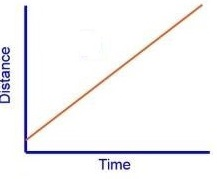(ii)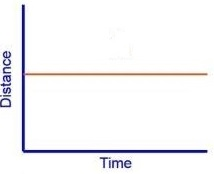Question 8. Which of the following relations is correct?
(i) Speed = Distance x Time.
(ii) Speed = $\frac{Distance}{Time}$
(iii) Speed = $\frac{Time}{Distance}$
(iv) Speed = $\frac{1}{Distance\phantom{\rule{thickmathspace}{0ex}}\phantom{\rule{thinmathspace}{0ex}}\phantom{\rule{thinmathspace}{0ex}}×\phantom{\rule{thickmathspace}{0ex}}Time}$
Answer: (ii) Speed = $\frac{Distance}{Time}$

Question 9. The basic unit of speed is:
(i) Km/min
(ii) m/min
(iii) km/h
(iv) m/s

Question 10. A car moves with a speed of 40 km/h for 15 minutes and then with a speed of
60 km/h for the next 15 minutes. The total distance covered by the car is :
(i) 100 km
(ii) 25 km
(iii) 15 km
(iv) 10 km

Question 11. Suppose the two photograph, shown in Fig. 13.1 and Fig.13.2, had been taken at an interval of 10 seconds. If a distance of 100 meters is shown by 1 cm in these photograph, calculate the speed of the blue car.

Answer: Speed = 100 m/10s = 10 m/s

Question 12. Fig. 13.5 shows the distance-time graph for the motion of two vehicles A and B. which is one of them moving faster?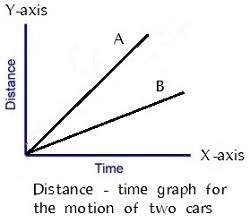Answer: Vehicle A is traveling longer distance in lesser time as compared to Vehicle B. So, vehicle A is moving faster.

Question 13. Which of the following distance time-graph a truck moving with speed which is not constant.